@import url(http://www.google.com/cse/api/branding.css);Custom Search

Fountain Products
Cave Surveying
 • Cave Survey Software
Free Utilities, Tools
 • Web Image Tracker • Sports Audio Delay • Time Calibrator • Spectrum Analyzer • Used Car Evaluater • Terrain Modeling • Free Topo Maps • XEdit Text Editor • EXPL Language
Fun Stuff
• Games
• Arachnid-32
• Eight-X Solitaire
Math, Computers
• 3D Mandelbrots
• Super-Spirograph
• 6502 Memorabilia

If you like our Programs Please Leave A TIP3D Mandelbrot Generator
 • Gallery  • 3D Manelbrot Algorithms • Marching Cubes Algorithm3D Mandelbrot Generator. I've attempted many times to create 3D Mandelbrot Sets with less than satisfying results. In the standard Mandelbrot Set, the iteration count is used to pick a color from a palette. One way to create a 3D Mandelbrot would be to use the iteration count as an elevation or z-axis value. This works reasonably well and with a little bit of work, you get images like the one to the right.

On the other hand, there is something unsatisfying about these images. While they are fractal in two dimensions, they are non-fractal and rather plain in the third dimension.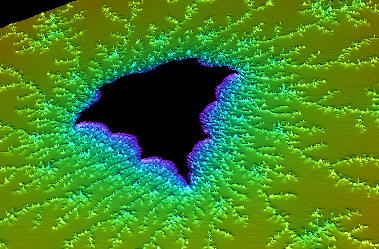2D Mandelbrot Set using color as the Z-dimension

Real 3D Mandelbrot. That all changed when I came across Daniel White's web page. Daniel's web page describes his own search for a true 3D Mandelbrot and his discoveries. The ultimate result is something that is now called the "Mandelbulb."

Daniel uses ray tracing to render his 3D Mandelbrots, but I thought it would be interesting to try OpenGL and 3D modeling. A 3D model would have the advantage that you could manipulate it in real time. The image to the right shows an example of what I was able to create.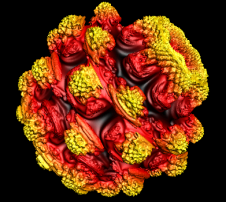True 3D Mandelbrot set
The Mandel Bulb

 Image Gallery Mandel Bulb Images3D Mandelbrot. - Degree = 4, Iterations = 6, Triangles = 1,502,808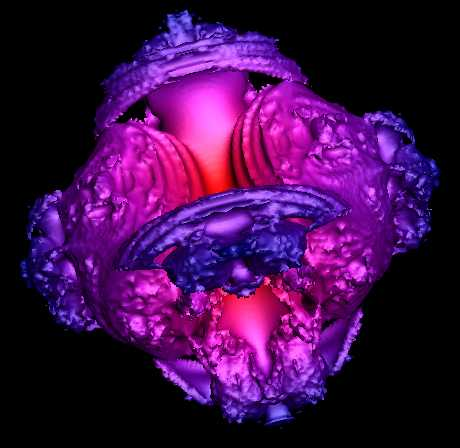3D Mandelbrot. - Degree = 3, Iterations = 5, Triangles = 251,0003D Mandelbrot. Degree = 32, Iterations = 2, Triangles = 12,720,0003D Mandelbrot. - Degree = 8, Iterations = 6, Triangles = 2,038,112
 Mandel Box ImagesMandel Box - Scale -1.7Mandel Box - Scale = 2.2  1,670,928 Triangles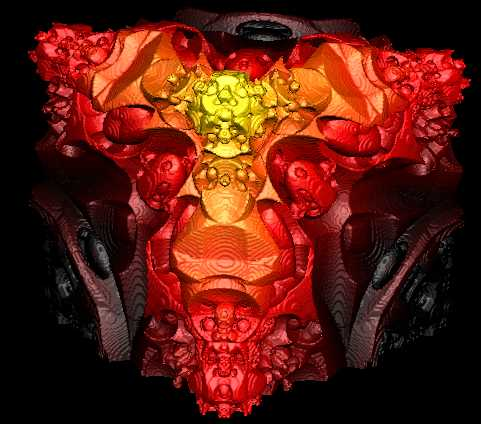Mandel Box - Scale = -1.7  2,931,245 TrianglesMandel Box - Scale = -2.1  Triangles = 640,735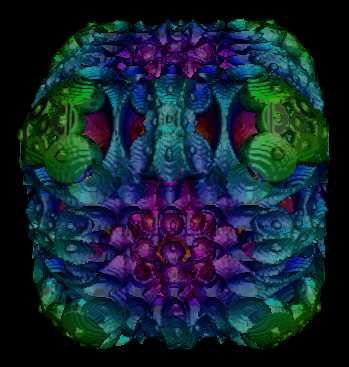Mandel Box - Scale = 2.2  Triangles = 1,784,248
3D Mandelbrot Algorithms and Math
 2D Mandelbrot 3D Mandelbrot  • 3D Surfaces  • Iterations/Degrees Mandel Box  • Box Fold  • Ball Fold
2D Mandelbrot.
The 3D Mandelbrot is similar to the 2D Mandelbrot, so it is useful to review how the 2D version works. In the 2D Mandelbrot, you are trying to find out if an X,Y point is a part of the Mandelbrot set. To do this, you plug the X,Y coordinates of the point into an equation that tests if the point is in the set. Points that are in the set are usually colored black; points that outside the set are colored according to how many iterations it took to find out it was outside the set.

The equation that tests a point looks like this: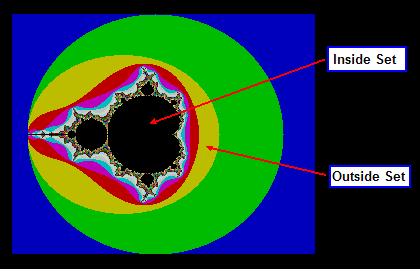Z' = Z^2 + C

This equation is processed repeatedly. Every time you process the equation, a new value of Z is calculated, which is designated Z'. This new point will be a certain distance from the center, or origin, of the image. If the distance of the new Z-value goes toward infinity, then the point we are testing is not part of the Mandelbrot set. We know that a value is going toward infinity if the distance exceeds two. Once it exceeds two, the distance will continue to get larger and larger and eventually go to infinity, so there is no need to test the point further.

Any point that is outside the set is colored according to how many iterations it took to discovered it was going to infinity. On the other hand, if the new value for Z never heads toward infinity, the point we are testing is part of the Mandelbrot set, and we color it black.

Complex Numbers. The one complication is that Z and C are complex numbers. In other words, Z and C have two components to each of them. For simplicity, we'll use vector notation and label them X and Y, so Z and C really look like this:

Z = Z.X + i*Z.Y
C = C.X + i*C.Y

This means the math operations are more complicated than they look:

 Z.X' = (Z.X * Z.X) + (Z.Y * Z.X) + (Z.X * Z.Y) - (Z.Y * Z.Y) + C.X Z.Y' = (Z.X * Z.X - Z.Y * Z.Y) + (Z.X * Z.Y + Z.Y * Z.X) + C.Y

We can simplify the operation algebraically so the expanded equations look like this:

Z.X' = Z.X^2 - Z.Y^2 + C.X
Z.Y' = 2 * Z.X * Z.Y + C.Y

The X and Y components of these complex numbers are used as the coordinates for points in the image. As a result, you can now plug the X,Y coordinates of the point you want to test into C.X and C.Y and then repeatedly process the equation to see what happens. Here is some Pascal code that performs the operations:

 ``` C.X:=X; C.Y:=Y; Z.X:=0; Z.Y:=0; for Count:=0 to ColorLimit-1 do begin if Sqrt(sqr(Z.X) + sqr(Z.Y)) > 2 then break; Z.Y:=2 * Z.Y * Z.X + C.Y; Z.X:=sqr(Z.X) - sqr(Z.Y) + C.X; end; if Count < ColorLimit then ColorPoint(X,Y,Colors[Count]); ```

The expression  Sqrt(sqr(Z.X) + sqr(Z.Y)) calculates the distance from the origin. If it exceeds 2, we know the point is not in the set, and we can quit without wasting any more time. Since we only color points that lie outside the set, the Count value gives us a way to choose a color. However, if the Count value reached the maximum number of colors without exceeding 2, the point must be in the set and we can leave the color black by not plotting it.

There are lots of ways to optimize the algorithm to improve its speed. For example, it is not necessary to calculate the actual distance. You can use the square-of-the-distance and eliminate the square root operation:

if (sqr(Z.X) + sqr(Z.Y))>4 then break;

3D Mandelbrot

Rotations. Daniel White's discovery is based on the fact that the 2D Mandelbrot can be thought of as a series of rotations on a plane. He thought that he might be able to find a satisfying 3D Mandelbrot if he extended the rotations to 3D. In other words, he began experimenting with rotations in a sphere. I won't go into the details here, but Daniel White does an excellent job of explaining this on his web page.

Here is the code that performs the 3D Mandelbrot:

```C.X:=Point.X;
C.Y:=Point.Y;
C.Z:=Point.Z;
for I:=0 to Iterations-1 do
begin
r := sqrt(C.x*C.x + C.y*C.y + C.z*C.z );
theta := arctan2(sqrt(C.x*C.x + C.y*C.y) , C.z);
phi := arctan2(C.y,C.x);
P:=IntPower(r,Degree);
New.x := P * sin(theta*Degree) * cos(phi*Degree);
New.y := P * sin(theta*Degree) * sin(phi*Degree);
New.z := P * cos(theta*Degree);
C:=VectorAdd3D(New,C);
Result:=sqrt(sqr(C.x)+sqr(C.y)+sqr(C.z));
if Result>1 then break;
end;```
As you can see, the algorithm is similar. As before, you plug a 3D coordinate of the point you wish to test into C and iterate through the equation until you exit. You can exit in two ways:

1) You may exit because C exceeds 1 indicating that the process is heading for infinity and there is no point in continuing. This indicates that the point is outside the surface of the 3D Mandelbrot.

2.) You may exit because you've hit the Iteration Limit without exceeding 1. This indicates the point is inside the surface of the Mandelbrot.

Sufaces. The big difference between the 2D and 3D Mandelbrots is that we are not interested in coloring points that sit outside the set. In this case, we are interested in displaying the 3D surface that precisely defines the boundary between points that are inside and outside the set. One way of doing this is with ray tracing.  If you are ray tracing, you simply test points along the path of the ray until the test shows you have hit the 3D surface.

Marching Cubes. Since I wanted to generate true 3D Surfaces, I needed to use a different technique. Generating a 3D mesh to model the surface is a bit complicated.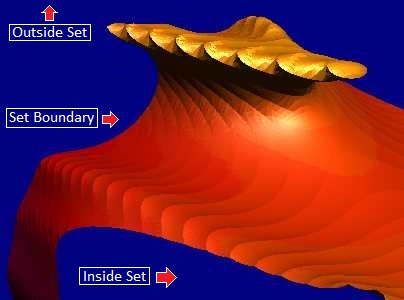You can't just test every point around the 3D Mandelbrot set. It would be computationally intensive and waste lots of time processing points that are no where near the set boundary.

The solution is to use the "Marching Cubes" algorithm. The algorithm divides the 3D space up into a series of cubes. You then test each cube to see if the surface falls inside the cube. If it does, you interpolate the location of the boundary and generate triangles from the points of intersection with the cubes. The smaller the cube, the more accurately the mesh approximates the surface, so you can easily adjust the resolution of the mesh by making the cubes bigger or smaller.

Degree and Iterations. If you look at the sample code above, you will see that the 3D Mandelbrot algorithm has two parameters that control its appearance: Degree and Iterations. The Degree variable controls the fundamental shape of the Mandelbrot. The Iterations variable controls the amount of the fractal complexity of the surface of the set.

The table and images below show how the Degree and Iterations interact to produce different fractals:

 Interations-1 Iterations = 4 Iterations = 6 Degree = 1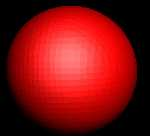Degree = 2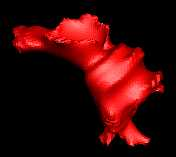Degree = 3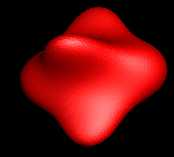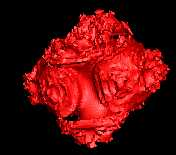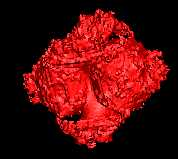Iterations = 1 Iterations = 2 Iterations = 5 Degree = 4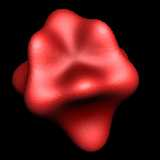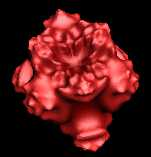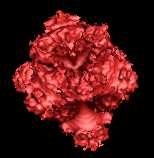Degree = 8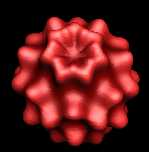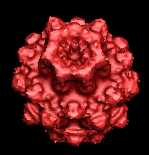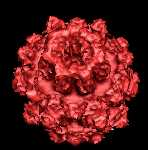Mandel Box
Mandel Box. The Mandel Box is another hyper-complex, 3D-Fractal invented by Tom Lowe in 2010, about a year after the Mandelbulb was discovered. It is similar to the other fractals, but uses folding and scaling operations to create a whole different genre of fractals. Here are some details:

The equation for the Mandel Box looks like this:

Z = BallFold( BoxFold(Z) ) * S + C

C = The 2D or 3D point that is being tested.
S = Floating point scaling value.
Z = A 2D/3D point that is initially set to zero.
BoxFold = A square folding function.
BallFold = A spherical folding function.

As before, the formula is iterated and tested to see if the Z-distance starts moving towards infinity.Folding. The key to the Mandel Box is a series of folding operations where the points are folded back on themselves in various ways.

Box Folds. The Box Folds fold the points back on themselves along straight lines. To create the fold, we check to see if a point is beyond the Fold Point and if it is, we fold it back by subtracting. For example, here is the code to do this for the X-Axis:

if X > 1 then X := 2 - X

The diagram to the right illustrates the process. If the X-value of a point were at 1.4, it would be folded back until it is repositioned at 0.6.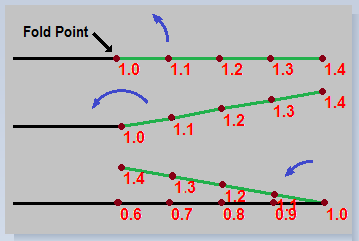The same process is performed on the negative X-Axis so that any point less than -1 is folded back toward the origin. Likewise, the same operation is performed on the Y- and Z-Axes. Taken together, the operation folds the points in a box-like pattern.

The animation to the right shows the folding operation on a 2D grid. You can see the grid being folded along the 1 and -1 lines for both the X and Y axis. (In the actual operation, the fold is carried out all at once, so you don't see the fold go through 180 degrees of rotation.

Here is sample code that performs the Box Fold: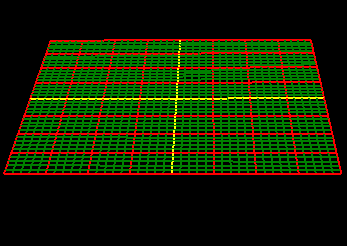` `
```procedure BoxFold(var Pnt: T3DVector);
begin
if Pnt.x > 1 then Pnt.x := 2 - Pnt.x
else if Pnt.x < -1 then Pnt.x := -2 - Pnt.x;

if Pnt.y > 1 then Pnt.y := 2 - Pnt.y
else if Pnt.y < -1 then Pnt.y := -2 - Pnt.y;

if Pnt.z > 1 then Pnt.z := 2 - Pnt.z
else if Pnt.z < -1 then Pnt.z := -2 - Pnt.z;
end;
```
Ball Fold. The next step is a more complex fold applied on top of the Box Fold. Instead of folding along a line, it is folded along the radius of two circles. The circles are designated by their radii. The larger circle is called the Fixed Radius and the smaller circle is called the Min Radius.

To accomplish the fold, all points inside the Min Radius are stretched so the inner radius is expanded out to 2. This is accomplished by multiplying each point inside the radius by 4. To see how the stretching is accomplished, here are examples of different radii multiplied by 4:

 0.5 X 4 = 2.0 0.4 x 4 = 1.6 0.3 x 4 = 1.2 0.2 x 4 = 0.8 0.1 x 4 = 0.4 0.0 x 4 = 0.0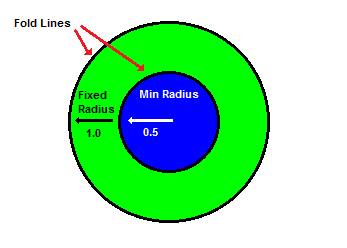In order to fold the points along both radii, the points between the Min Radius and Fixed Radius are flipped over and stretched. The diagram below shows the process. The blue line shows the points inside the Min Radius. The green line shows the points between the Min Radius and the Fixed Radius. The red line shows points beyond the Fixed Radius. As you can see in the bottom drawing, the blue line has been stretched and the green line has been both flipped and stretched.```const FixedRadius = 1.0;
const MinRadius = 0.5;
const RadiusRatio = sqr(FixedRadius) / sqr(MinRadius);

procedure BallFold(var Pnt: T3DVector);
var Radius,RF: double;
begin
Radius := sqrt(Sqr(Pnt.X) + Sqr(Pnt.Y) + Sqr(Pnt.Z));
if Radius < MinRadius then
begin
Pnt.X := Pnt.X * RadiusRatio;
Pnt.Y := Pnt.Y * RadiusRatio;
Pnt.Z := Pnt.Z * RadiusRatio;
end
else if Radius < FixedRadius then
begin
RF := Sqr(FixedRadius) / Sqr(Radius);
Pnt.X := Pnt.X * RF;
Pnt.Y := Pnt.Y * RF;
Pnt.Z := Pnt.Z * RF;
end
end;								```

The code above shows the Ball Fold operation. The code transforms one point, folding it as necessary. First, the distance from the origin is calculated, which gives you a Radius. If the radius is less than Minimum Radius, the point is multiplied by a constant, effectively stretching all points inside the inner circle. If the point is between Minimum Radius and Fixed Radius, the point is multiplied by a variable value that decreases as the radius approaches the Fixed Radius.

This effectively folds the points over the Fixed radius. Most people use constant values for the Fixed Radius and Minimum Radius of 1.0 and 0.5 respectively. Using those values, you can simplify the code a bit. RadioRatio becomes 4 and RF becomes 1/Radius^2.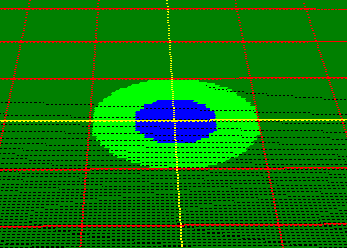The animation to the right shows the ball-fold process in two dimensions. The colored "bulls-eye" shows the two radii, with the blue circle showing the Minimum Radius and  the light-green circle showing the Fixed Radius.

Scaling.
The third step in the process is scaling. Each component of a point is multiplied by a scale factor. If the scale factor is greater than one, all the points are expanded away from the center. If the scale value is less than one, all the points contract toward the center.  Negative factors flips the points from positive to negative or negative to positive. The animation to the right illustrates the scaling process.

The animations show the folding and scaling in a smooth, continuous operation to visually illustrate the process. For example, the animations show the software slowly bending the points until the fold is completed. The actual folding and scaling process is carried out all at once.Escape. The folding and scaling operations are repeated for an individual point until it is clear whether the point is a part of the set. This determined by whether the point moves beyond a certain limit after several iterations.

The code to the right shows the process. After the Box Fold, Ball Fold and Scaling are done, the result is added to the test point C. Next, the magnitude of the new point is calculated. This will be the distance from the origin to the point. If the distance exceeds the Escape value, the point is outside the set. If the point makes it through all iterations without its distance exceeding the Escape value, the point is inside the set.

The full code for processing one point in the Mandel Box set is available here.

```
var Escape: integer = 4;
var Iterations: integer = 16;
```
```Point:=MakePoint(0,0,0);
Inside:=False;
for Iter:=0 to Iterations-1 do
begin
BoxFold(Point);
BallFold(Point);
Scale(Point);
Point:= VectorAdd(Point,C);
Len := Magnitude(Point);
if Len > Escape then exit;
end;
Inside:=True;```
 Scale Values. The most important parameter in the Mandelbox Algorithm is the Scale. Small changes in the scale, produce very different Mandelbrot sets. Negative values also produce interesting effects. Experimenting with different scales can produce surprisingly different results. The images to the right show Mandelbrots sets derived from different scales. The top image shows a Mandelbox generated with a scale of 2. The bottom image shows a scale of -3. Another commonly used scaled is -1.7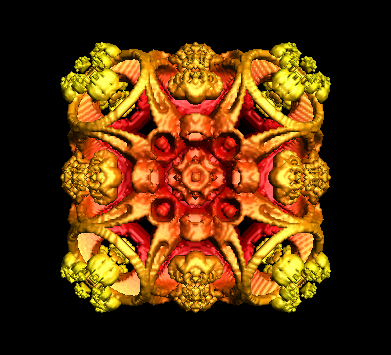Scale = 2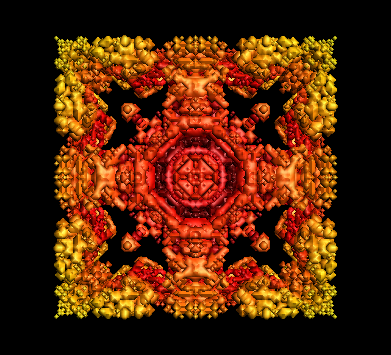Scale = -3

 Contact UsCustom Search